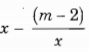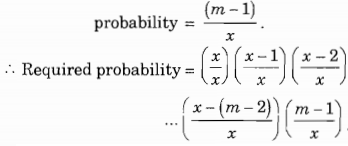Data Structures and Algorithms - Performance Analysis of Algorithms and Recurrences

> > > > > > > > Performance Analysis of Algorithms and Recurrences

• Option : A
• Explanation :
Probability for the first record not colliding is x/x.
Probability for the second record not colliding is x-1/x.
(This is because one place is already occupied, So, favorable number of cases is x-1).
Probability for the third record not colliding is x-2/x.
Probability for the (m-1)th record not colliding is.
Now the next (mth) record is resulting in a collision. Out of the x places, it should has to one of the (m-1) places already, filled. So,• A

Linear programming• B

Travelling Salesmen Problem• C

Presburger arithmetic• D

Hamiltonian circuit problem• A

n2• B

n• C

n3• D

nn• Option : B
• Explanation :
Recursively applying the relation, we finally get
T(n + 1) = c(n - 1) + T(1)
= c(n - 1) + d
hence order is n.

• A

O(log n) < O(n) < O( n* log n) < O(2n) < O(n2)• B

O(n) < O(log n) < O( n* log n) < O(2n) < O(n2)• C

O(n) < O(log n) < O( n* log n) < O(n2) < O(2n)• D

O(log n) < O(n) < O( n* log n) < O(n2) < O(2n)• A

F is O(n7) but not O(n6)• B

F is O(n6) but not O(n5)• C

F is O(n5) but not O(n4)• D

F is O(n4) but not O(n3)